(Affiliate Notice: G-Central may earn a commission on sales made from its links to Amazon, eBay, and Reeds.)

# G-Shock MTGG1000D-1A2 gets U.S. release

`The G-Shock MTGG1000D-1A2 from the new Metal Twisted G-Shock (MT-G) MTG-G1000 line is officially getting a U.S. release in October. The MTGG1000 is the third G-Shock with the GPS Atomic Solar Hybrid feature. This technology uses both Multi-Band 6 radio and GPS satellite signal reception for automatic time adjustment anywhere in the world, ensuring the watch is always accurate no matter the location. The watch also automatically determines its timezone and DST setting. `

``` The MTGG1000D-1A2 has a unique core guard structure in which a fine resin core housing the module is situated between two metal frames and is surrounded by Alpha Gel for advanced shock protection. It has Triple G Resist for protection against shock, centrifugal g-force, and heavy vibration. A new feature of the MTG-G1000 series is the high-torque, dual-coil motors that can quickly adjust the world time subdial from home time to local time. The hour, minute, and second hands are made of carbon fiber and allow for quicker hand movements. Other luxury features include sapphire crystal with anti-glare coating, composite band made of stainless steel and resin, high quality 3D markers and Sallaz Polishing for a mirror-like finish on certain areas. The watch is solar powered with a rechargeable battery. It also has a latitude indicator, airplane mode, LED super-illuminator, dual dial world time with 40 cities + UTC, daily alarm, auto calendar, stopwatch (24 hour), countdown timer (24 hour), and day/date display. The MTGG1000D-1A2 has a stainless steel exterior with a blue dial ring and blue accents on the face. It has a list price of \$1,500 and will be available at G-Shock, the G-Shock Soho Store, and select jewelers. The MTG-G1000 series is also available in two models as an import. MTGG1000D-1A2 on Amazon MTGG1000D-1A2 on eBay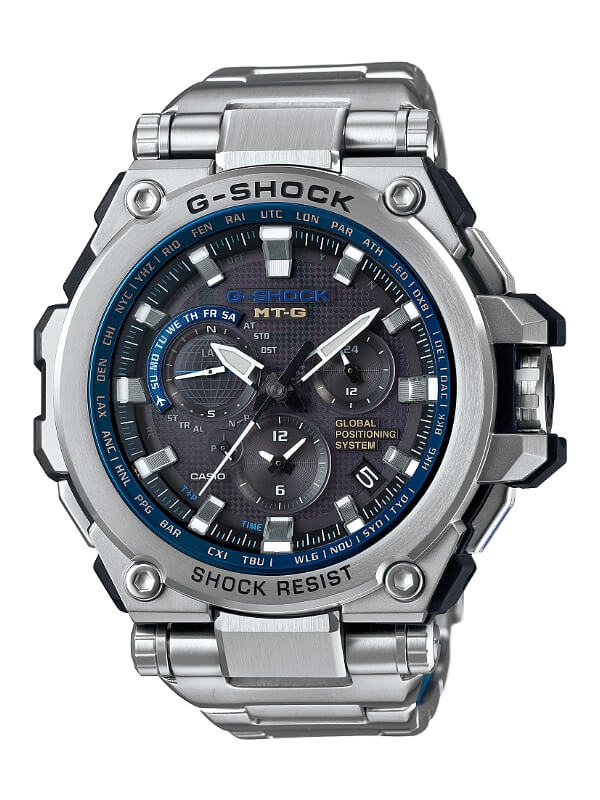jQuery(document).ready(function(\$){\$.post('https://www.g-central.com/wp-admin/admin-ajax.php',{action:'mts_view_count',id:'4776'});});Share This Post (Disclosure: G-Central is independently managed by fans and is not an official G-Shock site. For official G-Shock support, visit world.casio.com. As an Amazon Associate, eBay Partner, and Reeds Jewelers affiliate, G-Central earns on qualifying purchases, at no additional cost to the customer. ) Shop for new releases and see our top picks at: amazon.com/shop/gcentralhq Click Here for the Latest G-Shocks at Authorized Dealer Reeds Jewelers (adsbygoogle=window.adsbygoogle||[]).push({}); ```
``` ```
``` ```
``` Tags:metal, MT-G, MTG-G1000, sapphire, Triple G Resist ```
``` Leave a Reply document.getElementById("comment").setAttribute("id","adf582758959bb3cab1ed77e0ce39a73");document.getElementById("f8f5aea9b5").setAttribute("id","comment"); Save my name, email, and website in this browser for the next time I comment. ```
``` ```
``` FEATURED WATCHES (G-Central may earn a commission) amazon.com/shop/gcentralhqCurated by G-Central⌚ See limited time deals on men's watches hereAdvertisements: (adsbygoogle=window.adsbygoogle||[]).push({});Latest TweetG-Central G-Shock Fan Blog@gcentralblog·19 OctWe added more images of the Five Tigers General series by Jahan Loh and the Dazzling Night (black-orange-blue) series https://www.g-central.com/Twitter 1318097111859236864Load More...New G-Shocks For Sale (“Featured” Category)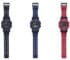More G-Shock GA-900 Colors: Black (1A), Blue (2A), Red (4A)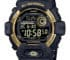Black and Gold G-Shock G-8900GB-1: Unusual but welcome for 2020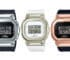G-Shock GM-S5600: A Smaller Metal (S Series) Square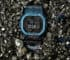G-Shock GMW-B5000TCF-2: Titanium with Blue IP Camouflage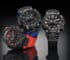G-Shock GR-B200 Gravitymaster has a Quad Sensor and Carbon Core Guard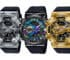G-Shock GM-110 is a metalized GA-110 with stainless steel bezel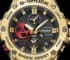Rui Hachimura x G-Shock GST-B100RH-1A Signature Model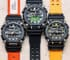The G-Shock GA-900 has a heavy-duty industrial style with a decagonal bezel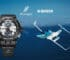HondaJet x G-Shock GWR-B1000HJ-1A Gravitymaster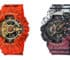Dragon Ball Z and One Piece x G-Shock Collaborations for 2020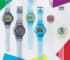G-Shock Color Skeleton Series with Fluorescent Accents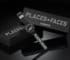PLACES+FACES x G-Shock DW-6900PF-1 Collaboration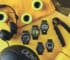G-Shock Digital Camouflage Series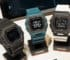G-Shock G-LIDE GBX-100 with MIP LCD, Step Counter, Tide, Vibe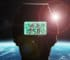NASA x G-Shock DW5600NASA20-7CR “All Systems Go” Collab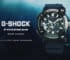G-Shock Frogman GWF-A1000 with Full Analog Display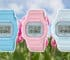G-Shock DW-5600SC Spring Color Pastel Series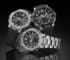G-Shock G-STEEL GST-B300 with Front Button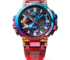G-Shock MT-G MTG-B1000VL-4A Volcanic Lightning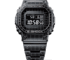G-Shock GMW-B5000CS-1 with Laser-Engraved Grid Pattern Popular Posts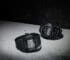G-Shock GWM5610-1 Versus GW6900-1: What’s the difference? …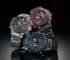G-Shock MTG-B2000 Dual Core Guard Carbon Monocoque …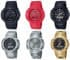AW-500 and AWM-500: Revival of First Analog …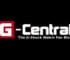G-Talk: October 2020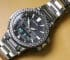Pro Trek PRX-8025HT-1JR for Pro Trek 25th … ```
``` About Us: G-Central is a fan-managed site covering the latest G-Shock watch releases and news.  Coverage includes worldwide releases, regional releases, limited editions, collaborations, events, and more.  Our articles are regularly updated to include new information and active shopping links.  G-Central is independently owned and operated and is not an official representative of G-Shock or Casio.  G-Shock is a registered trademark of Casio Computer Co., Ltd. Visit the official Casio G-Shock sites at world.g-shock.com. Shop • FAQ • Guides • RSS • Support Us • ContactEmail Subscription Affiliate Disclosure: As an Amazon Associate I earn from qualifying purchases. G-Central may also earn on sales from its links to eBay and Reeds Jewelers.Cookie Notice: G-Central uses cookies to personalize content, provide social media features, serve ads, and analyze traffic. By using this site, you consent to the use of cookies, or you may choose to disable them in your browser settings. See full disclosure G-Central G-Shock Watch Fan Blog Copyright © 2020. Privacy Policy & Disclosure  ```
``` (function(i,s,o,g,r,a,m){i['GoogleAnalyticsObject']=r;i[r]=i[r]||function(){(i[r].q=i[r].q||[]).push(arguments)},i[r].l=1*new Date();a=s.createElement(o),m=s.getElementsByTagName(o);a.async=1;a.src=g;m.parentNode.insertBefore(a,m)})(window,document,'script','https://www.g-central.com/wp-content/cache/busting/google-tracking/ga-1e3ad19b0836d257e66df0e4106af582.js','ga');ga('create','UA-60191359-1','auto');ga('send','pageview'); //<![CDATA[ var ctf={"ajax_url":"https:\/\/www.g-central.com\/wp-admin\/admin-ajax.php"}; //]]> G-Central uses cookies to personalize ads, provide social media features, and analyze traffic. Click 'Okay' to consent to the use of cookies.Okay, I AcceptLearn More //<![CDATA[ ai_front={"insertion_before":"BEFORE","insertion_after":"AFTER","insertion_prepend":"PREPEND CONTENT","insertion_append":"APPEND CONTENT","insertion_replace_content":"REPLACE CONTENT","insertion_replace_element":"REPLACE ELEMENT","visible":"VISIBLE","hidden":"HIDDEN","fallback":"FALLBACK","automatically_placed":"Automatically placed by AdSense Auto ads code","cancel":"Cancel","use":"Use","add":"Add","parent":"Parent","cancel_element_selection":"Cancel element selection","select_parent_element":"Select parent element","css_selector":"CSS selector","use_current_selector":"Use current selector","element":"ELEMENT","path":"PATH","selector":"SELECTOR"};function b2a(a){var b,c=0,l=0,f="",g=[];if(!a)return a;do{var e=a.charCodeAt(c++);var h=a.charCodeAt(c++);var k=a.charCodeAt(c++);var d=e<<16|h<<8|k;e=63&d>>18;h=63&d>>12;k=63&d>>6;d&=63;g[l++]="ABCDEFGHIJKLMNOPQRSTUVWXYZabcdefghijklmnopqrstuvwxyz0123456789+/=".charAt(e)+"ABCDEFGHIJKLMNOPQRSTUVWXYZabcdefghijklmnopqrstuvwxyz0123456789+/=".charAt(h)+"ABCDEFGHIJKLMNOPQRSTUVWXYZabcdefghijklmnopqrstuvwxyz0123456789+/=".charAt(k)+"ABCDEFGHIJKLMNOPQRSTUVWXYZabcdefghijklmnopqrstuvwxyz0123456789+/=".charAt(d)}while(c<a.length);return f=g.join(""),b=a.length%3,(b?f.slice(0,b-3):f)+"===".slice(b||3)}function a2b(a){var b,c,l,f={},g=0,e=0,h="",k=String.fromCharCode,d=a.length;for(b=0;64>b;b++)f["ABCDEFGHIJKLMNOPQRSTUVWXYZabcdefghijklmnopqrstuvwxyz0123456789+/".charAt(b)]=b;for(c=0;d>c;c++)for(b=f[a.charAt(c)],g=(g<<6)+b,e+=6;8<=e;)((l=255&g>>>(e-=8))||d-2>c)&&(h+=k(l));return h}b64e=function(a){return btoa(encodeURIComponent(a).replace(/%([0-9A-F]{2})/g,function(b,a){return String.fromCharCode("0x"+a)}))};b64d=function(a){return decodeURIComponent(atob(a).split("").map(function(a){return"%"+("00"+a.charCodeAt(0).toString(16)).slice(-2)}).join(""))};var \$jscomp=\$jscomp||{};\$jscomp.scope={};\$jscomp.arrayIteratorImpl=function(a){var b=0;return function(){return b<a.length?{done:!1,value:a[b++]}:{done:!0}}};\$jscomp.arrayIterator=function(a){return{next:\$jscomp.arrayIteratorImpl(a)}};\$jscomp.makeIterator=function(a){var b="undefined"!=typeof Symbol&&Symbol.iterator&&a[Symbol.iterator];return b?b.call(a):\$jscomp.arrayIterator(a)};(function(a){if("function"===typeof define&&define.amd){define(a);var b=!0}"object"===typeof exports&&(module.exports=a(),b=!0);if(!b){var f=window.Cookies,c=window.Cookies=a();c.noConflict=function(){window.Cookies=f;return c}}})(function(){function a(){for(var a=0,c={};a<arguments.length;a++){var b=arguments[a],e;for(e in b)c[e]=b[e]}return c}function b(f){function c(){}function d(b,e,d){if("undefined"!==typeof document){d=a({path:"/"},c.defaults,d);"number"===typeof d.expires&&(d.expires=new Date(1*new Date+864E5*d.expires));d.expires=d.expires?d.expires.toUTCString():"";try{var g=JSON.stringify(e);/^[\{\[]/.test(g)&&(e=g)}catch(n){}e=f.write?f.write(e,b):encodeURIComponent(String(e)).replace(/%(23|24|26|2B|3A|3C|3E|3D|2F|3F|40|5B|5D|5E|60|7B|7D|7C)/g,decodeURIComponent);b=encodeURIComponent(String(b)).replace(/%(23|24|26|2B|5E|60|7C)/g,decodeURIComponent).replace(/[\(\)]/g,escape);g="";for(var k in d)d[k]&&(g+="; "+k,!0!==d[k]&&(g+="="+d[k].split(";")));return document.cookie=b+"="+e+g}}function e(a,b){if("undefined"!==typeof document){for(var c={},e=document.cookie?document.cookie.split("; "):[],d=0;d<e.length;d++){var g=e[d].split("="),h=g.slice(1).join("=");b||'"'!==h.charAt(0)||(h=h.slice(1,-1));try{var l=g.replace(/(%[0-9A-Z]{2})+/g,decodeURIComponent);h=(f.read||f)(h,l)||h.replace(/(%[0-9A-Z]{2})+/g,decodeURIComponent);if(b)try{h=JSON.parse(h)}catch(m){}c[l]=h;if(a===l)break}catch(m){}}return a?c[a]:c}}c.set=d;c.get=function(a){return e(a,!1)};c.getJSON=function(a){return e(a,!0)};c.remove=function(b,c){d(b,"",a(c,{expires:-1}))};c.defaults={};c.withConverter=b;return c}return b(function(){})});AiCookies=Cookies.noConflict();ai_check_block=function(a){if(null==a)return!0;var b=AiCookies.getJSON("aiBLOCKS");ai_debug_cookie_status="";null==b&&(b={});"undefined"!==typeof ai_delay_showing_pageviews&&(b.hasOwnProperty(a)||(b[a]={}),b[a].hasOwnProperty("d")||(b[a].d=ai_delay_showing_pageviews));if(b.hasOwnProperty(a))for(var f in b[a]){if("x"==f){var c="",d=document.querySelectorAll('span[data-ai-block="'+a+'"]');"aiHash"in d.dataset&&(c=d.dataset.aiHash);d="";b[a].hasOwnProperty("h")&&(d=b[a].h);var e=new Date;e=b[a][f]-Math.round(e.getTime()/1E3);if(0<e&&d==c)return ai_debug_cookie_status=a="closed for "+e+" s = "+Math.round(1E4*e/3600/24)/1E4+" days",!1;ai_set_cookie(a,"x","");b[a].hasOwnProperty("i")||b[a].hasOwnProperty("c")||ai_set_cookie(a,"h","")}else if("d"==f){if(0!=b[a][f])return ai_debug_cookie_status=a="delayed for "+b[a][f]+" pageviews",!1}else if("i"==f){c="";d=document.querySelectorAll('span[data-ai-block="'+a+'"]');"aiHash"in d.dataset&&(c=d.dataset.aiHash);d="";b[a].hasOwnProperty("h")&&(d=b[a].h);if(0==b[a][f]&&d==c)return ai_debug_cookie_status=a="max impressions reached",!1;if(0>b[a][f]&&d==c){e=new Date;e=-b[a][f]-Math.round(e.getTime()/1E3);if(0<e)return ai_debug_cookie_status=a="max imp. reached ("+Math.round(1E4*e/24/3600)/1E4+" days = "+e+" s)",!1;ai_set_cookie(a,"i","");b[a].hasOwnProperty("c")||b[a].hasOwnProperty("x")||ai_set_cookie(a,"h","")}}if("ipt"==f&&0==b[a][f]&&(e=new Date,c=Math.round(e.getTime()/1E3),e=b[a].it-c,0<e))return ai_debug_cookie_status=a="max imp. per time reached ("+Math.round(1E4*e/24/3600)/1E4+" days = "+e+" s)",!1;if("c"==f){c="";d=document.querySelectorAll('span[data-ai-block="'+a+'"]');"aiHash"in d.dataset&&(c=d.dataset.aiHash);d="";b[a].hasOwnProperty("h")&&(d=b[a].h);if(0==b[a][f]&&d==c)return ai_debug_cookie_status=a="max clicks reached",!1;if(0>b[a][f]&&d==c){e=new Date;e=-b[a][f]-Math.round(e.getTime()/1E3);if(0<e)return ai_debug_cookie_status=a="max clicks reached ("+Math.round(1E4*e/24/3600)/1E4+" days = "+e+" s)",!1;ai_set_cookie(a,"c","");b[a].hasOwnProperty("i")||b[a].hasOwnProperty("x")||ai_set_cookie(a,"h","")}}if("cpt"==f&&0==b[a][f]&&(e=new Date,c=Math.round(e.getTime()/1E3),e=b[a].ct-c,0<e))return ai_debug_cookie_status=a="max clicks per time reached ("+Math.round(1E4*e/24/3600)/1E4+" days = "+e+" s)",!1}ai_debug_cookie_status="OK";return!0};ai_check_and_insert_block=function(a,b){if(null==a)return!0;var f=document.getElementsByClassName(b);if(f.length){f=f;var c=f.closest(".code-block");if(ai_check_block(a))ai_insert_code(f),c&&(c.classList.remove("ai-list-block"),c.style.visibility="",c.classList.contains("ai-remove-position")&&(c.style.position=""));else{var d=f.closest("div[data-ai]");if(null!=d&&"undefined"!=typeof d.getAttribute("data-ai")){var e=JSON.parse(b64d(d.getAttribute("data-ai")));"undefined"!==typeof e&&e.constructor===Array&&(e="",d.setAttribute("data-ai",b64e(JSON.stringify(e))))}d=c.querySelectorAll(".ai-debug-block");c&&d.length&&(c.classList.remove("ai-list-block"),c.style.visibility="",c.classList.contains("ai-remove-position")&&(c.style.position=""))}f.classList.remove(b)}f=document.querySelectorAll("."+b+"-dbg");f=\$jscomp.makeIterator(f);for(c=f.next();!c.done;c=f.next())c=c.value,c.querySelector(".ai-status").textContent=ai_debug_cookie_status,c.querySelector(".ai-cookie-data").textContent=ai_get_cookie_text(a),c.classList.remove(b+"-dbg")};function ai_load_cookie(){var a=AiCookies.getJSON("aiBLOCKS");null==a&&(a={});return a}function ai_get_cookie(a,b){var f="",c=ai_load_cookie();c.hasOwnProperty(a)&&c[a].hasOwnProperty(b)&&(f=c[a][b]);return f}function ai_set_cookie(a,b,f){var c=ai_load_cookie();if(""===f){if(c.hasOwnProperty(a)){delete c[a][b];a:{b=c[a];for(d in b)if(b.hasOwnProperty(d)){var d=!1;break a}d=!0}d&&delete c[a]}}else c.hasOwnProperty(a)||(c[a]={}),c[a][b]=f;0===Object.keys(c).length&&c.constructor===Object?AiCookies.remove("aiBLOCKS"):AiCookies.set("aiBLOCKS",c,{expires:365,path:"/"});return c}ai_get_cookie_text=function(a){var b=AiCookies.getJSON("aiBLOCKS");null==b&&(b={});return b.hasOwnProperty(a)?JSON.stringify(b[a]).replace(/"/g,"").replace("{","").replace("}",""):""};ai_insert=function(b,l,u){var r=-1!=l.indexOf(":eq")?jQuery(l):document.querySelectorAll(l);Array.prototype.forEach.call(r,function(g,w){selector_string=g.hasAttribute("id")?"#"+g.getAttribute("id"):g.hasAttribute("class")?"."+g.getAttribute("class").replace(RegExp(" ","g"),"."):"";var k=document.createElement("div");k.innerHTML=u;var m=k.getElementsByClassName("ai-selector-counter");null!=m&&(m.innerText=w+1);m=k.getElementsByClassName("ai-debug-name ai-main");if(null!=m){var t="";"before"==b?t=ai_front.insertion_before:"after"==b?t=ai_front.insertion_after:"prepend"==b?t=ai_front.insertion_prepend:"append"==b?t=ai_front.insertion_append:"replace-content"==b?t=ai_front.insertion_replace_content:"replace-element"==b&&(t=ai_front.insertion_replace_element);-1==selector_string.indexOf(".ai-viewports")&&(m.innerText=t+" "+l+" ("+g.tagName.toLowerCase()+selector_string+")")}m=document.createRange().createContextualFragment(k.innerHTML);"before"==b?g.parentNode.insertBefore(m,g):"after"==b?g.parentNode.insertBefore(m,g.nextSibling):"prepend"==b?g.insertBefore(m,g.firstChild):"append"==b?g.insertBefore(m,null):"replace-content"==b?g.innerHTML=k.innerHTML:"replace-element"==b&&(g.parentNode.insertBefore(m,g),g.parentNode.removeChild(g))})};ai_insert_code=function(b){function l(m,t){return null==m?!1:m.classList?m.classList.contains(t):-1<(" "+m.className+" ").indexOf(" "+t+" ")}function u(m,t){null!=m&&(m.classList?m.classList.add(t):m.className+=" "+t)}function r(m,t){null!=m&&(m.classList?m.classList.remove(t):m.className=m.className.replace(new RegExp("(^|\\b)"+t.split(" ").join("|")+"(\\b|\$)","gi")," "))}if("undefined"!=typeof b){var g=!1;if(l(b,"no-visibility-check")||b.offsetWidth||b.offsetHeight||b.getClientRects().length){g=b.getAttribute("data-code");var w=b.getAttribute("data-insertion"),k=b.getAttribute("data-selector");if(null!=g)if(null!=w&&null!=k){if(-1!=k.indexOf(":eq")?jQuery(k).length:document.querySelectorAll(k).length)ai_insert(w,k,b64d(g)),r(b,"ai-viewports")}else g=document.createRange().createContextualFragment(b64d(g)),b.parentNode.insertBefore(g,b.nextSibling),r(b,"ai-viewports");g=!0;b=b.getElementsByClassName("ai-check-block");"undefined"!=typeof b&&b.parentNode.removeChild(b)}else w=b.previousElementSibling,l(w,"ai-debug-bar")&&l(w,"ai-debug-script")&&(r(w,"ai-debug-script"),u(w,"ai-debug-viewport-invisible")),r(b,"ai-viewports");return g}};ai_insert_list_code=function(b){var l=document.getElementsByClassName(b);if("undefined"!=typeof l){var u=ai_insert_code(l),r=l.closest("div.code-block");r&&(u||r.removeAttribute("data-ai"),r.classList.remove("ai-list-block"),r.style.visibility="",r.classList.contains("ai-remove-position")&&(r.style.position=""));l.classList.remove(b);u&&ai_process_elements()}};ai_insert_viewport_code=function(b){var l=document.getElementsByClassName(b);"undefined"!=typeof l&&(ai_insert_code(l),l.classList.remove(b),ai_process_elements())};ai_insert_code_by_class=function(b){var l=document.getElementsByClassName(b);"undefined"!=typeof l&&(ai_insert_code(l),l.classList.remove(b))};ai_insert_client_code=function(b,l){var u=document.getElementsByClassName(b);if("undefined"!=typeof u){var r=u.getAttribute("data-code");null!=r&&ai_check_block()&&ai_check_and_insert_block()&&(u.setAttribute("data-code",r.substring(Math.floor(l/19))),ai_insert_code_by_class(b),u.remove())}};ai_process_elements_active=!1;function ai_process_elements(){ai_process_elements_active||setTimeout(function(){ai_process_elements_active=!1;"function"==typeof ai_process_rotations&&ai_process_rotations();"function"==typeof ai_process_lists&&ai_process_lists(jQuery("div.ai-list-data"));"function"==typeof ai_process_ip_addresses&&ai_process_ip_addresses(jQuery("div.ai-ip-data"));"function"==typeof ai_adb_process_blocks&&ai_adb_process_blocks()},5);ai_process_elements_active=!0}var Arrive=function(b,l,u){function r(q,c,d){k.addMethod(c,d,q.unbindEvent);k.addMethod(c,d,q.unbindEventWithSelectorOrCallback);k.addMethod(c,d,q.unbindEventWithSelectorAndCallback)}function g(q){q.arrive=x.bindEvent;r(x,q,"unbindArrive");q.leave=y.bindEvent;r(y,q,"unbindLeave")}if(b.MutationObserver&&"undefined"!==typeof HTMLElement){var w=0,k=function(){var q=HTMLElement.prototype.matches||HTMLElement.prototype.webkitMatchesSelector||HTMLElement.prototype.mozMatchesSelector||HTMLElement.prototype.msMatchesSelector;return{matchesSelector:function(c,d){return c instanceof HTMLElement&&q.call(c,d)},addMethod:function(c,d,e){var a=c[d];c[d]=function(){if(e.length==arguments.length)return e.apply(this,arguments);if("function"==typeof a)return a.apply(this,arguments)}},callCallbacks:function(c,d){d&&d.options.onceOnly&&1==d.firedElems.length&&(c=[c]);for(var e=0,a;a=c[e];e++)a&&a.callback&&a.callback.call(a.elem,a.elem);d&&d.options.onceOnly&&1==d.firedElems.length&&d.me.unbindEventWithSelectorAndCallback.call(d.target,d.selector,d.callback)},checkChildNodesRecursively:function(c,d,e,a){for(var f=0,h;h=c[f];f++)e(h,d,a)&&a.push({callback:d.callback,elem:h}),0<h.childNodes.length&&k.checkChildNodesRecursively(h.childNodes,d,e,a)},mergeArrays:function(c,d){var e={},a;for(a in c)c.hasOwnProperty(a)&&(e[a]=c[a]);for(a in d)d.hasOwnProperty(a)&&(e[a]=d[a]);return e},toElementsArray:function(c){"undefined"===typeof c||"number"===typeof c.length&&c!==b||(c=[c]);return c}}}(),m=function(){var q=function(){this._eventsBucket=[];this._beforeRemoving=this._beforeAdding=null};q.prototype.addEvent=function(c,d,e,a){c={target:c,selector:d,options:e,callback:a,firedElems:[]};this._beforeAdding&&this._beforeAdding(c);this._eventsBucket.push(c);return c};q.prototype.removeEvent=function(c){for(var d=this._eventsBucket.length-1,e;e=this._eventsBucket[d];d--)c(e)&&(this._beforeRemoving&&this._beforeRemoving(e),(e=this._eventsBucket.splice(d,1))&&e.length&&(e.callback=null))};q.prototype.beforeAdding=function(c){this._beforeAdding=c};q.prototype.beforeRemoving=function(c){this._beforeRemoving=c};return q}(),t=function(q,c){var d=new m,e=this,a={fireOnAttributesModification:!1};d.beforeAdding(function(f){var h=f.target;if(h===b.document||h===b)h=document.getElementsByTagName("html");var n=new MutationObserver(function(v){c.call(this,v,f)});var p=q(f.options);n.observe(h,p);f.observer=n;f.me=e});d.beforeRemoving(function(f){f.observer.disconnect()});this.bindEvent=function(f,h,n){h=k.mergeArrays(a,h);for(var p=k.toElementsArray(this),v=0;v<p.length;v++)d.addEvent(p[v],f,h,n)};this.unbindEvent=function(){var f=k.toElementsArray(this);d.removeEvent(function(h){for(var n=0;n<f.length;n++)if(this===u||h.target===f[n])return!0;return!1})};this.unbindEventWithSelectorOrCallback=function(f){var h=k.toElementsArray(this);d.removeEvent("function"===typeof f?function(n){for(var p=0;p<h.length;p++)if((this===u||n.target===h[p])&&n.callback===f)return!0;return!1}:function(n){for(var p=0;p<h.length;p++)if((this===u||n.target===h[p])&&n.selector===f)return!0;return!1})};this.unbindEventWithSelectorAndCallback=function(f,h){var n=k.toElementsArray(this);d.removeEvent(function(p){for(var v=0;v<n.length;v++)if((this===u||p.target===n[v])&&p.selector===f&&p.callback===h)return!0;return!1})};return this},x=new function(){function q(e,a,f){return k.matchesSelector(e,a.selector)&&(e._id===u&&(e._id=w++),-1==a.firedElems.indexOf(e._id))?(a.firedElems.push(e._id),!0):!1}var c={fireOnAttributesModification:!1,onceOnly:!1,existing:!1};x=new t(function(e){var a={attributes:!1,childList:!0,subtree:!0};e.fireOnAttributesModification&&(a.attributes=!0);return a},function(e,a){e.forEach(function(f){var h=f.addedNodes,n=f.target,p=[];null!==h&&0<h.length?k.checkChildNodesRecursively(h,a,q,p):"attributes"===f.type&&q(n,a,p)&&p.push({callback:a.callback,elem:n});k.callCallbacks(p,a)})});var d=x.bindEvent;x.bindEvent=function(e,a,f){"undefined"===typeof f?(f=a,a=c):a=k.mergeArrays(c,a);var h=k.toElementsArray(this);if(a.existing){for(var n=[],p=0;p<h.length;p++)for(var v=h[p].querySelectorAll(e),z=0;z<v.length;z++)n.push({callback:f,elem:v[z]});if(a.onceOnly&&n.length)return f.call(n.elem,n.elem);setTimeout(k.callCallbacks,1,n)}d.call(this,e,a,f)};return x},y=new function(){function q(e,a){return k.matchesSelector(e,a.selector)}var c={};y=new t(function(){return{childList:!0,subtree:!0}},function(e,a){e.forEach(function(f){f=f.removedNodes;var h=[];null!==f&&0<f.length&&k.checkChildNodesRecursively(f,a,q,h);k.callCallbacks(h,a)})});var d=y.bindEvent;y.bindEvent=function(e,a,f){"undefined"===typeof f?(f=a,a=c):a=k.mergeArrays(c,a);d.call(this,e,a,f)};return y};l&&g(l.fn);g(HTMLElement.prototype);g(NodeList.prototype);g(HTMLCollection.prototype);g(HTMLDocument.prototype);g(Window.prototype);l={};r(x,l,"unbindAllArrive");r(y,l,"unbindAllLeave");return l}}(window,"undefined"===typeof jQuery?null:jQuery,void 0);jQuery(function(a){function q(){a("div.ai-rotate.ai-rotation-groups").each(function(b,k){a(this).addClass("ai-timer");ai_process_rotation(this)})}var l=[];ai_process_rotation=function(b){if(a(b).hasClass("ai-unprocessed")||a(b).hasClass("ai-timer"))if(a(b).removeClass("ai-unprocessed").removeClass("ai-timer"),l.includes(b.selector)&&l.splice(l.indexOf(b.selector),1),"number"==typeof b.length)for(var k=0;k<b.length;k++)0==k?ai_process_single_rotation(b[k],!0):ai_process_single_rotation(b[k],!1);else ai_process_single_rotation(b,!0)};ai_process_single_rotation=function(b,k){var h=a(b).children(".ai-rotate-option");if(0!=h.length){h.hide();if("undefined"==typeof a(b).data("next"))if("undefined"!=typeof a(h).data("group")){var g=-1,p=[];a("span[data-ai-groups]").each(function(b){(a(this).offsetWidth||a(this).offsetHeight||a(this).getClientRects().length)&&p.push(this)});1<=p.length&&JSON.parse(b64d(a(p).first().data("ai-groups"))).forEach(function(b,c){-1==g&&h.each(function(c){if(b64d(a(this).data("group"))==b)return g=c,!1})})}else{var e=a(b).data("shares");if("string"===typeof e){e=JSON.parse(atob(e));var f=Math.round(100*Math.random());for(var c=0;c<e.length&&(g=c,0>e[c]||!(f<=e[c]));c++);}else e=a(b).hasClass("ai-unique"),f=new Date,e?("number"!=typeof ai_rotation_seed&&(ai_rotation_seed=(Math.floor(1E3*Math.random())+f.getMilliseconds())%h.length),e=a(b).data("counter"),g=ai_rotation_seed+e,g>=h.length&&(g-=h.length)):(g=Math.floor(Math.random()*h.length),f.getMilliseconds()%2&&(g=h.length-g-1))}else g=parseInt(a(b).attr("data-next")),f=a(h[g]),"undefined"!=typeof f.data("code")&&(f=a(b64d(f.data("code")))),0!=f.find("span[data-ai-groups]").addBack("span[data-ai-groups]").length&&0!=a(".ai-rotation-groups").length&&setTimeout(function(){q()},5);if(!(0>g||g>=h.length)){f=a(h[g]);e="";if("undefined"!=typeof f.data("time")){c=atob(f.data("time"));if(0==c&&1<h.length){var d=g;do{d++;d>=h.length&&(d=0);var m=a(h[d]);if("undefined"==typeof m.data("time")){g=d;f=a(h[g]);c=0;break}m=atob(m.data("time"))}while(0==m&&d!=g);0!=c&&(g=d,f=a(h[g]),c=atob(f.data("time")))}if(0<c&&(d=g+1,d>=h.length&&(d=0),"undefined"!=typeof a(b).data("info"))){e=JSON.parse(atob(a(b).data("info")));a(b).attr("data-next",d);var n="div.ai-rotate.ai-"+e;l.includes(n)&&(k=!1);k&&(l.push(n),setTimeout(function(){a(n).addClass("ai-timer");ai_process_rotation(a(n))},1E3*c));e=" ("+c+" s)"}}else"undefined"==typeof f.data("group")&&h.each(function(b){b!=g&&a(this).remove()});f.css({display:"",visibility:"",position:"",width:"",height:"",top:"",left:""}).removeClass("ai-rotate-hidden").removeClass("ai-rotate-hidden-2");a(b).css({position:""});"undefined"!=typeof f.data("code")&&(h.empty(),c=b64d(f.data("code")),f.append(c),ai_process_elements());f=b64d(f.data("name"));d=a(b).closest(".ai-debug-block");0!=d.length&&(c=d.find("kbd.ai-option-name"),d=d.find(".ai-debug-block"),"undefined"!=typeof d&&(d=d.find("kbd.ai-option-name"),c=c.slice(0,c.length-d.length)),"undefined"!=typeof c&&(d=c.first().data("separator"),"undefined"==typeof d&&(d=""),c.html(d+f+e)));c=!1;d=a(b).closest(".ai-adb-show");0!=d.length&&d.attr("data-ai-tracking")&&(e=JSON.parse(b64d(d.attr("data-ai-tracking"))),"undefined"!==typeof e&&e.constructor===Array&&(e=g+1,e=f,d.attr("data-ai-tracking",b64e(JSON.stringify(e))),c=!0));c||(c=a(b).closest("div[data-ai]"),"undefined"!=typeof c.attr("data-ai")&&(e=JSON.parse(b64d(c.attr("data-ai"))),"undefined"!==typeof e&&e.constructor===Array&&(e=g+1,e=f,c.attr("data-ai",b64e(JSON.stringify(e))))))}}};ai_process_rotations=function(){a("div.ai-rotate").each(function(a,k){ai_process_rotation(this)})};ai_process_rotations_in_element=function(b){a("div.ai-rotate",b).each(function(a,b){ai_process_rotation(this)})};a(document).ready(function(a){setTimeout(function(){ai_process_rotations()},10)})});ai_process_elements_active=!1;function ai_process_elements(){ai_process_elements_active||setTimeout(function(){ai_process_elements_active=!1;"function"==typeof ai_process_rotations&&ai_process_rotations();"function"==typeof ai_process_lists&&ai_process_lists(jQuery("div.ai-list-data"));"function"==typeof ai_process_ip_addresses&&ai_process_ip_addresses(jQuery("div.ai-ip-data"));"function"==typeof ai_adb_process_blocks&&ai_adb_process_blocks()},5);ai_process_elements_active=!0};var \$jscomp=\$jscomp||{};\$jscomp.scope={};\$jscomp.createTemplateTagFirstArg=function(a){return a.raw=a};\$jscomp.createTemplateTagFirstArgWithRaw=function(a,k){a.raw=k;return a};\$jscomp.arrayIteratorImpl=function(a){var k=0;return function(){return k<a.length?{done:!1,value:a[k++]}:{done:!0}}};\$jscomp.arrayIterator=function(a){return{next:\$jscomp.arrayIteratorImpl(a)}};\$jscomp.makeIterator=function(a){var k="undefined"!=typeof Symbol&&Symbol.iterator&&a[Symbol.iterator];return k?k.call(a):\$jscomp.arrayIterator(a)};jQuery(function(a){function k(h){h=h.match(p);return null!=h&&1<h.length&&"string"===typeof h&&0<h.length?h.toLowerCase():null}function y(h){try{var f=Date.parse(h);isNaN(f)&&(f=null)}catch(z){f=null}return f}Array.prototype.includes||(Array.prototype.includes=function(h){return!!~this.indexOf(h)});var p=/:\/\/(.[^/:]+)/i;ai_process_lists=function(h){function f(d,b,g){if(0==d.length){if("!@!"==g)return!0;b!=g&&("true"==g.toLowerCase()?g=!0:"false"==g.toLowerCase()&&(g=!1));return b==g}if("object"!=typeof b&&"array"!=typeof b)return!1;var e=d;d=d.slice(1);if("*"==e)for(b=\$jscomp.makeIterator(Object.entries(b)),e=b.next();!e.done;e=b.next()){if(e=\$jscomp.makeIterator(e.value),e.next(),e=e.next().value,f(d,e,g))return!0}else if(e in b)return f(d,b[e],g);return!1}function z(d,b,g){if("object"!=typeof d||-1==b.indexOf("["))return!1;b=b.replace(/]| /gi,"").split("[");return f(b,d,g)}function T(){"function"==typeof __tcfapi&&(a("#ai-iab-tcf-status").text("DETECTED"),__tcfapi("getTCData",2,function(d,b){b?(a("#ai-iab-tcf-bar").addClass("status-ok"),"tcloaded"==d.eventStatus||"useractioncomplete"==d.eventStatus?(ai_tcData=d,d.gdprApplies?a("#ai-iab-tcf-status").text("DATA LOADED"):jQuery("#ai-iab-tcf-status").text("GDPR DOES NOT APPLY"),a("#ai-iab-tcf-bar").addClass("status-ok").removeClass("status-error"),setTimeout(function(){ai_process_lists()},10)):"cmpuishown"==d.eventStatus&&(ai_cmpuishown=!0,a("#ai-iab-tcf-status").text("CMP UI SHOWN"),a("#ai-iab-tcf-bar").addClass("status-ok").removeClass("status-error"))):(a("#ai-iab-tcf-status").text("__tcfapi getTCData failed"),a("#ai-iab-tcf-bar").removeClass("status-ok").addClass("status-error"))}))}function K(d){"function"==typeof __tcfapi?"undefined"==typeof ai_tcData_requested&&(ai_tcData_requested=!0,T(),url_parameters_need_tcData=!0):d&&(a("#ai-iab-tcf-bar").addClass("status-error").removeClass("status-ok"),a("#ai-iab-tcf-status").text("MISSING: __tcfapi function not found"))}h=null==h?a("div.ai-list-data"):h.filter(".ai-list-data");if(h.length){h.removeClass("ai-list-data");var C=document.cookie.split(";");C.forEach(function(d,b){C[b]=d.trim()});var L=getAllUrlParams(window.location.search);if(null!=L.referrer)var q=L.referrer;else q=document.referrer,""!=q&&(q=k(q));var F=window.navigator.userAgent,G=F.toLowerCase();if("undefined"!==typeof MobileDetect)var M=new MobileDetect(F);h.each(function(){var d=a(this).closest("div.code-block"),b=!0,g=a(this).attr("referer-list");if("undefined"!=typeof g){g=b64d(g).split(",");var e=a(this).attr("referer-list-type"),m=!1;a.each(g,function(u,c){if(""==c)return!0;if("*"==c.charAt(0))if("*"==c.charAt(c.length-1)){if(c=c.substr(1,c.length-2),-1!=q.indexOf(c))return m=!0,!1}else{if(c=c.substr(1),q.substr(-c.length)==c)return m=!0,!1}else if("*"==c.charAt(c.length-1)){if(c=c.substr(0,c.length-1),0==q.indexOf(c))return m=!0,!1}else if("#"==c){if(""==q)return m=!0,!1}else if(c==q)return m=!0,!1});switch(e){case"B":m&&(b=!1);break;case"W":m||(b=!1)}}if(b&&(g=a(this).attr("client-list"),"undefined"!=typeof g&&"undefined"!==typeof M))switch(g=b64d(g).split(","),e=a(this).attr("client-list-type"),m=!1,a.each(g,function(u,c){if(""==c)return!0;if("*"==c.charAt(0))if("*"==c.charAt(c.length-1)){if(c=c.substr(1,c.length-2).toLowerCase(),-1!=G.indexOf(c))return m=!0,!1}else{if(c=c.substr(1).toLowerCase(),G.substr(-c.length)==c)return m=!0,!1}else if("*"==c.charAt(c.length-1)){if(c=c.substr(0,c.length-1).toLowerCase(),0==G.indexOf(c))return m=!0,!1}else if(M.is(c))return m=!0,!1}),e){case"B":m&&(b=!1);break;case"W":m||(b=!1)}var N=g=!1;if(b&&(e=a(this).attr("parameter-list"),"undefined"!=typeof e)){e=b64d(e).split(",");var r=a(this).attr("parameter-list-type"),O=[];C.forEach(function(u){u=u.split("=");try{var c=JSON.parse(decodeURIComponent(u))}catch(P){c=decodeURIComponent(u)}O[u]=c});var v=!1,D=a(this);a.each(e,function(u,c){var P=c.split("&&");a.each(P,function(V,n){var w=!0;n=n.trim();"!!"==n.substring(0,2)&&(w=!1,n=n.substring(2));var t=n,x="!@!",Q=-1!=n.indexOf("["),R=0==n.indexOf("euconsent-v2")&&-1!=n.indexOf("[");-1!=n.indexOf("=")&&(x=n.split("="),t=x,x=x,Q=-1!=t.indexOf("["),R=0==t.indexOf("euconsent-v2")&&-1!=t.indexOf("["));if(R)a("#ai-iab-tcf-bar").show(),"object"==typeof ai_tcData?(a("#ai-iab-tcf-bar").addClass("status-ok"),t=t.replace(/]| /gi,"").split("["),t.shift(),v=(t=f(t,ai_tcData,x))?w:!w):(D.addClass("ai-list-data"),N=!0,"function"==typeof __tcfapi?K(!1):"undefined"==typeof ai_tcData_retrying&&(ai_tcData_retrying=!0,setTimeout(function(){K(!0)},200)));else if(Q)v=(t=z(O,t,x))?w:!w;else{var H=!1;"!@!"==x?C.every(function(U){return U.split("=")==n?(H=!0,!1):!0}):H=-1!=C.indexOf(n);v=H?w:!w}if(!v)return!1});if(v)return!1});switch(r){case"B":v&&(b=!1);break;case"W":v||(b=!1)}a(this).hasClass("ai-list-manual")&&(b?(D.removeClass("ai-list-data"),D.removeClass("ai-list-manual")):(g=!0,D.addClass("ai-list-data")));e=a(this).data("debug-info");if("undefined"!=typeof e&&(e=a("."+e),0!=e.length)){var l=e.parent();l.hasClass("ai-debug-info")&&l.remove()}}l=a(this).prevAll(".ai-debug-bar.ai-debug-lists");e=""==q?"#":q;l.find(".ai-debug-name.ai-list-info").text(e).attr("title",F);l.find(".ai-debug-name.ai-list-status").text(b?ai_front.visible:ai_front.hidden);e=!1;if(b){var E=a(this).attr("scheduling-start");l=a(this).attr("scheduling-end");var A=a(this).attr("scheduling-days");if("undefined"!=typeof E&&"undefined"!=typeof l&&"undefined"!=typeof A){e=!0;var I=parseInt(a(this).attr("scheduling-fallback"));r=parseInt(a(this).attr("gmt"));var B=y(b64d(E))+r;l=y(b64d(l))+r;E=b64d(A).split(",");A=a(this).attr("scheduling-type");var J=(new Date).getTime()+r,S=new Date(J);r=S.getDay();0==r?r=6:r--;B=J>=B&&J<l&&E.includes(r.toString());switch(A){case"B":B=!B}B||(b=!1);A=S.toISOString().split(".").replace("T"," ");l=a(this).prevAll(".ai-debug-bar.ai-debug-scheduling");l.find(".ai-debug-name.ai-scheduling-info").text(A+" "+r);l.find(".ai-debug-name.ai-scheduling-status").text(b?ai_front.visible:ai_front.hidden);b||0==I||(l.removeClass("ai-debug-scheduling").addClass("ai-debug-fallback"),l.find(".ai-debug-name.ai-scheduling-status").text(ai_front.fallback+"="+I))}}if(g||N)return!0;a(this).css({visibility:"",position:"",width:"",height:"","z-index":""});b?(d.css({visibility:""}),d.hasClass("ai-remove-position")&&d.css({position:""}),"undefined"!=typeof a(this).data("code")&&(b=b64d(a(this).data("code")),a(this).append(b),ai_process_element(this))):e&&!B&&0!=I?(d.css({visibility:""}),d.hasClass("ai-remove-position")&&d.css({position:""}),a(this).next(".ai-fallback").removeClass("ai-fallback"),"undefined"!=typeof a(this).data("fallback-code")?(b=b64d(a(this).data("fallback-code")),a(this).append(b),ai_process_element(this)):a(this).hide(),b=d.attr("data-ai"),"undefined"!==typeof b&&!1!==b&&(b=a(this).attr("fallback-tracking"),"undefined"!==typeof b&&!1!==b&&d.attr("data-ai",b))):(a(this).hide(),d.removeAttr("data-ai").removeClass("ai-track"),d.find(".ai-debug-block").length?(d.css({visibility:""}).removeClass("ai-close"),d.hasClass("ai-remove-position")&&d.css({position:""})):d.hide());a(this).attr("data-code","");a(this).attr("data-fallback-code","");d.removeClass("ai-list-block")})}};a(document).ready(function(h){setTimeout(function(){ai_process_lists();(jQuery("#ai-iab-tcf-bar").length||jQuery(".ai-list-manual").length)&&"function"==typeof __tcfapi&&"function"==typeof ai_load_blocks&&__tcfapi("addEventListener",2,function(f,z){z&&"useractioncomplete"===f.eventStatus&&(ai_tcData=f,ai_load_blocks(),jQuery("#ai-iab-tcf-status").text("DATA LOADED"),jQuery("#ai-iab-tcf-bar").addClass("status-ok").removeClass("status-error"))});if("function"==typeof ai_load_blocks)jQuery(document).on("cmplzEnableScripts",function(f){"all"===f.consentLevel&&ai_load_blocks()});jQuery("#ai-iab-tcf-bar").click(function(){AiCookies.remove("euconsent-v2",{path:"/",domain:"."+window.location.hostname});jQuery("#ai-iab-tcf-status").text("COOKIE DELETED")})},5)})});function ai_process_element(a){setTimeout(function(){"function"==typeof ai_process_rotations_in_element&&ai_process_rotations_in_element(a);"function"==typeof ai_process_lists&&ai_process_lists(jQuery("div.ai-list-data",a));"function"==typeof ai_process_ip_addresses&&ai_process_ip_addresses(jQuery("div.ai-ip-data",a));"function"==typeof ai_adb_process_blocks&&ai_adb_process_blocks(a)},5)}function getAllUrlParams(a){var k=a?a.split("?"):window.location.search.slice(1);a={};if(k){k=k.split("#");k=k.split("&");for(var y=0;y<k.length;y++){var p=k[y].split("="),h=void 0,f=p.replace(/\[\d*\]/,function(z){h=z.slice(1,-1);return""});p="undefined"===typeof p?"":p;f=f.toLowerCase();p=p.toLowerCase();a[f]?("string"===typeof a[f]&&(a[f]=[a[f]]),"undefined"===typeof h?a[f].push(p):a[f][h]=p):a[f]=p}}return a};jQuery(function(b){function m(b,c){c||(c=window.location.href);b=b.replace(/[\[\]]/g,"\\\$&");var a=(new RegExp("[?&]"+b+"(=([^&#]*)|&|#|\$)")).exec(c);return a?a?decodeURIComponent(a.replace(/\+/g," ")):"":null}function n(d){d.removeClass("ai-ip-data");var c=!1;if(""!=ai_ip_data){try{var a=JSON.parse(ai_ip_data),h=a,p=a,g=a,k=a}catch(q){return}var l=h+", "+p;null!=g&&null!=k&&(l=l+":"+g+":"+k);null==g&&(g="");null==k&&(k="");null!=a&&d.each(function(){var a=b(this).closest("div.code-block");c=!0;var e=b(this).attr("ip-addresses");if("undefined"!=typeof e){e=e.split(",");var d=b(this).attr("ip-address-list"),f=!1;b.each(e,function(b,a){if("*"==a.charAt(0))if("*"==a.charAt(a.length-1)){if(a=a.substr(1,a.length-2),-1!=h.indexOf(a))return f=!0,!1}else{if(a=a.substr(1),h.substr(-a.length)==a)return f=!0,!1}else if("*"==a.charAt(a.length-1)){if(a=a.substr(0,a.length-1),0==h.indexOf(a))return f=!0,!1}else if("#"==a){if(""==h)return f=!0,!1}else if(a==h)return f=!0,!1});switch(d){case"B":f&&(c=!1);break;case"W":f||(c=!1)}}if(c&&(e=b(this).attr("countries"),"undefined"!=typeof e))switch(e=e.split(","),d=b(this).attr("country-list"),f=!1,b.each(e,function(a,b){var c=b.trim().split(":");if(null==c||""==g)c="";if(null==c||""==k)c="";var d=c.join(":").toUpperCase();c=(p+":"+(""==c?"":g)+":"+(""==c?"":k)).toUpperCase();if(d==c)return f=!0,!1}),d){case"B":f&&(c=!1);break;case"W":f||(c=!1)}b(this).css({visibility:"",position:"",width:"",height:"","z-index":""});e=b(this).prev(".ai-debug-bar");e.find(".ai-debug-name.ai-ip-country").text(l);e.find(".ai-debug-name.ai-ip-status").text(c?ai_front.visible:ai_front.hidden);c?(a.css({visibility:""}),a.hasClass("ai-remove-position")&&a.css({position:""}),"undefined"!=typeof b(this).data("code")&&(e=b64d(b(this).data("code")),b(this).append(e),b(this).attr("data-code",""),ai_process_element(this))):(b(this).hide(),a.removeAttr("data-ai"),a.find(".ai-debug-block")?(a.css({visibility:""}).removeClass("ai-close"),a.hasClass("ai-remove-position")&&a.css({position:""})):a.hide());a.removeClass("ai-list-block")})}}ai_process_ip_addresses=function(d){d=null==d?b("div.ai-ip-data"):d.filter(".ai-ip-data");if(d.length)if(d.removeClass("ai-ip-data"),"undefined"!=typeof ai_ip_data)n(d);else{var c="/wp-admin/admin-ajax.php?action=ai_ajax&ip-data=ip-address-country-city&ai_check=028043bb3f&ver="+((new Date).getTime()+"-"+Math.round(1E5*Math.random())),a=m("ai-debug-ip-address");null!=a&&(c+="&ai-debug-ip-address="+a);a=m("ai-debug-country");null!=a&&(c+="&ai-debug-country="+a);b.get(c,function(a){ai_ip_data=a;if(""==a){var b="Ajax request returned empty data, geo-targeting disabled";console.error(b);"undefined"!=typeof ai_js_errors&&ai_js_errors.push([b,c,0])}else try{JSON.parse(a)}catch(g){b="Ajax call returned invalid data, geo-targeting disabled",console.error(b,a),"undefined"!=typeof ai_js_errors&&ai_js_errors.push([b,c,0])}n(d)}).fail(function(a,c,d){b("div.ai-ip-data").each(function(){b(this).css({display:"none",visibility:"",position:"",width:"",height:"","z-index":""}).removeClass("ai-ip-data").hide()})})}};b(document).ready(function(b){setTimeout(function(){ai_process_ip_addresses()},5)})});function ai_process_element(b){setTimeout(function(){"function"==typeof ai_process_rotations_in_element&&ai_process_rotations_in_element(b);"function"==typeof ai_process_lists&&ai_process_lists(jQuery("div.ai-list-data",b));"function"==typeof ai_process_ip_addresses&&ai_process_ip_addresses(jQuery("div.ai-ip-data",b));"function"==typeof ai_adb_process_blocks&&ai_adb_process_blocks(b)},5)};ai_js_code=true; //]]> ```